Next: Approximate string matching Up: Sequence comparison Previous: Basic Problem

# Levenshtein Distance

If the following values are considered:
• Sub(a,a) = 0 for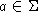;
• Sub(a,b) = 1 for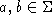and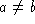;
• Del(a) = Ins(a) = 1 for.
Then T[m-1,n-1] represents the Levenshtein distance () between x and y.

Example :
x = YHCQPGK and y = LAHYQQKPGKA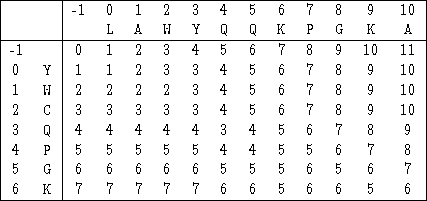This gives the six following alignments: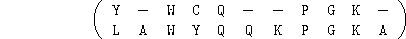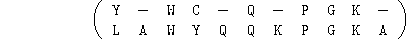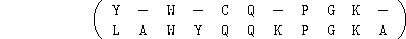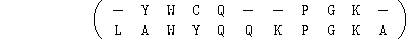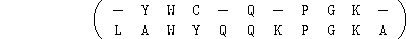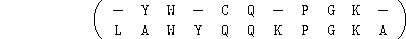which correspond to the six minimum cost paths between (-1,-1) and (6,10):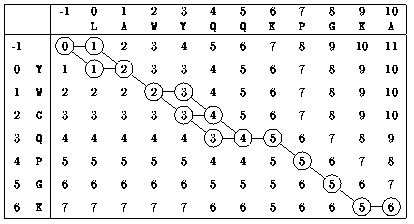Next: Approximate string matching Up: Sequence comparison Previous: Basic Problem

e-mails: {Christian.Charras, Thierry.Lecroq}@laposte.net
Thu Feb 19 10:23:29 WET 1998